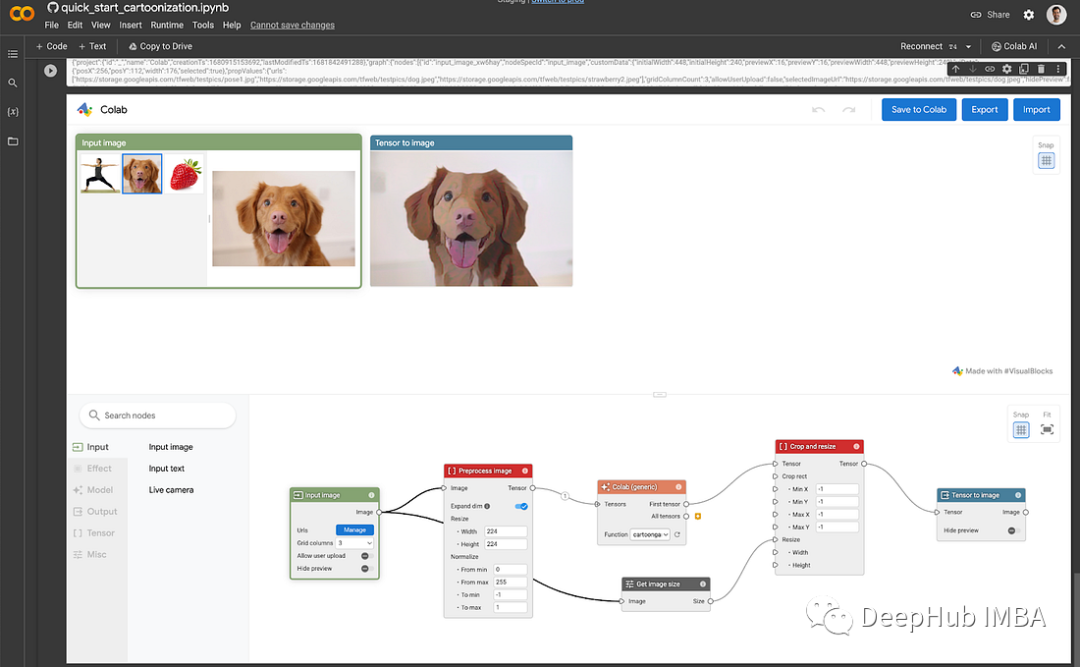#### Python-np.expand_dims()

Python-np.expand_dims()用于扩展数组的维度。执行程序后注意观察中括号[ ]的位置和数量

#### 【AI人工智能】如何使用Keras和TensorFlow来训练大型深度学习模型

Keras和TensorFlow都使用了动态图(Dynamic Graph)作为模型的表示。动态图允许模型在运行时进行修改，并且可以在编译时进行优化。Keras和TensorFlow都使用了神经网络模型的压缩和优化技术。例如，Keras的Transformer模型采用了一些针对压缩和优化的技术，例如

#### tensorflow如何使用gpu

tensorflow查看GPU的数量、使用GPU加速，单GPU模拟多GPU环境等

#### 查看cudnn&cuda的版本以及对应的tensorflow版本#### 深入浅出TensorFlow2函数——tf.rank

tf.rank(input, name=None)

#### 深入浅出TensorFlow2函数——tf.exp

tf.exp( x, name=None)

#### 深入浅出TensorFlow2函数——tf.random.uniform

tf.random.uniform( shape, minval=0, maxval=None, dtype=tf.dtypes.float32, seed=None, name=None)

#### 深入浅出TensorFlow2函数——tf.data.Dataset.from_tensor_slices

from_tensor_slices( tensors, name=None)

tf.random.normal

#### 深入浅出TensorFlow2函数——tf.constant

tf.constant( value, dtype=None, shape=None, name='Const')

#### 深入浅出TensorFlow2函数——tf.random.poisson

tf.random.poisson(shape,lam,dtype=tf.dtypes.float32,seed=None, name=None)

#### 深入浅出TensorFlow2函数——tf.math.exp

tf.math.exp( x, name=None)

#### 深入浅出TensorFlow2函数——tf.Tensor##### 作者榜##### 资讯小助手##### 内容小助手##### Deephub